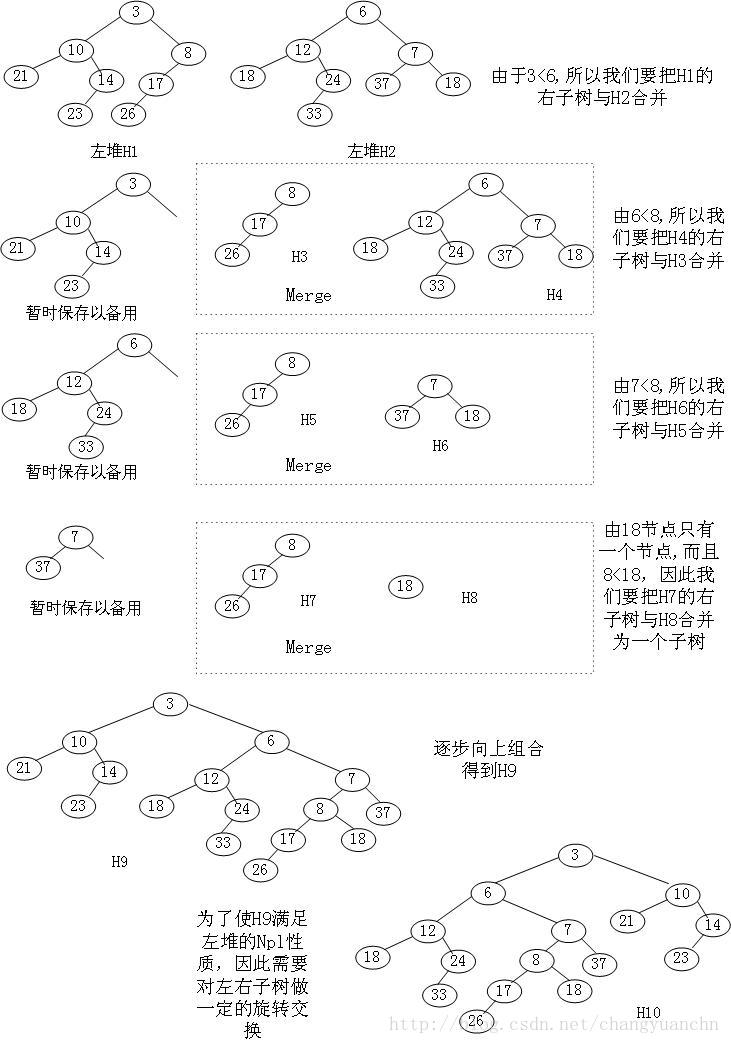左偏堆 | Kyons'Blog

## 三.基本概念

$Npl(X)$：

## 四.合并## 例题

### (hdu 1512) Monkey King

http://acm.hdu.edu.cn/showproblem.php?pid=1512

#### Problem Description

Once in a forest, there lived $N$ aggressive monkeys. At the beginning, they each does things in its own way and none of them knows each other. But monkeys can’t avoid quarrelling, and it only happens between two monkeys who does not know each other. And when it happens, both the two monkeys will invite the strongest friend of them, and duel. Of course, after the duel, the two monkeys and all of there friends knows each other, and the quarrel above will no longer happens between these monkeys even if they have ever conflicted.
Assume that every money has a strongness value, which will be reduced to only half of the original after a duel(that is, $10$ will be reduced to $5$ and $5$ will be reduced to $2$).
And we also assume that every monkey knows himself. That is, when he is the strongest one in all of his friends, he himself will go to duel.

#### Input

There are several test cases, and each case consists of two parts.
First part: The first line contains an integer $N$($N\leq100000$), which indicates the number of monkeys. And then $N$ lines follows. There is one number on each line, indicating the strongness value of ith monkey($\leq32768$).
Second part: The first line contains an integer $M$($M\leq100000$), which indicates there are $M$ conflicts happened. And then $M$ lines follows, each line of which contains two integers $x$ and $y$, indicating that there is a conflict between the $X_{th}$ monkey and $Y_{th}$.

#### Output

For each of the conflict, output $-1$ if the two monkeys know each other, otherwise output the strongness value of the strongest monkey in all friends of them after the duel.
Sample Input
5
20
16
10
10
4
5
2 3
3 4
3 5
4 5
1 5
Sample Output
8
5
5
-1
10
Author
JIANG, Yanyan
Source
ZOJ 3rd Anniversary Contest
Recommend
linle

#### 解析//删除Valine核心代码库外链调用 //调用刚下载的本地文件以加速加载速度 //这里改为从本地加载